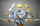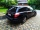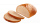# Fraction calculator

The calculator performs basic and advanced operations with fractions, expressions with fractions combined with integers, decimals, and mixed numbers. It also shows detailed step-by-step information about the fraction calculation procedure. Solve problems with two, three, or more fractions and numbers in one expression.

## Result:

### 7/9 + 2/3 = 13/9 = 1 4/9 ≅ 1.4444444

Spelled result in words is thirteen ninths (or one and four ninths).

### How do you solve fractions step by step?

1. Add: 7/9 + 2/3 = 7/9 + 2 · 3/3 · 3 = 7/9 + 6/9 = 7 + 6/9 = 13/9
For adding, subtracting, and comparing fractions, it is suitable to adjust both fractions to a common (equal, identical) denominator. The common denominator you can calculate as the least common multiple of both denominators - LCM(9, 3) = 9. In practice, it is enough to find the common denominator (not necessarily the lowest) by multiplying the denominators: 9 × 3 = 27. In the following intermediate step, the fraction result cannot be further simplified by canceling.
In other words - seven ninths plus two thirds = thirteen ninths.

#### Rules for expressions with fractions:

Fractions - use the slash “/” between the numerator and denominator, i.e., for five-hundredths, enter 5/100. If you are using mixed numbers, be sure to leave a single space between the whole and fraction part.
The slash separates the numerator (number above a fraction line) and denominator (number below).

Mixed numerals (mixed fractions or mixed numbers) write as non-zero integer separated by one space and fraction i.e., 1 2/3 (having the same sign). An example of a negative mixed fraction: -5 1/2.
Because slash is both signs for fraction line and division, we recommended use colon (:) as the operator of division fractions i.e., 1/2 : 3.

Decimals (decimal numbers) enter with a decimal point . and they are automatically converted to fractions - i.e. 1.45.

The colon : and slash / is the symbol of division. Can be used to divide mixed numbers 1 2/3 : 4 3/8 or can be used for write complex fractions i.e. 1/2 : 1/3.
An asterisk * or × is the symbol for multiplication.
Plus + is addition, minus sign - is subtraction and ()[] is mathematical parentheses.
The exponentiation/power symbol is ^ - for example: (7/8-4/5)^2 = (7/8-4/5)2

#### Examples:

subtracting fractions: 2/3 - 1/2
multiplying fractions: 7/8 * 3/9
dividing Fractions: 1/2 : 3/4
exponentiation of fraction: 3/5^3
fractional exponents: 16 ^ 1/2
adding fractions and mixed numbers: 8/5 + 6 2/7
dividing integer and fraction: 5 ÷ 1/2
complex fractions: 5/8 : 2 2/3
decimal to fraction: 0.625
Fraction to Decimal: 1/4
Fraction to Percent: 1/8 %
comparing fractions: 1/4 2/3
multiplying a fraction by a whole number: 6 * 3/4
square root of a fraction: sqrt(1/16)
reducing or simplifying the fraction (simplification) - dividing the numerator and denominator of a fraction by the same non-zero number - equivalent fraction: 4/22
expression with brackets: 1/3 * (1/2 - 3 3/8)
compound fraction: 3/4 of 5/7
fractions multiple: 2/3 of 3/5
divide to find the quotient: 3/5 ÷ 2/3

The calculator follows well-known rules for order of operations. The most common mnemonics for remembering this order of operations are:
PEMDAS - Parentheses, Exponents, Multiplication, Division, Addition, Subtraction.
BEDMAS - Brackets, Exponents, Division, Multiplication, Addition, Subtraction
BODMAS - Brackets, Of or Order, Division, Multiplication, Addition, Subtraction.
GEMDAS - Grouping Symbols - brackets (){}, Exponents, Multiplication, Division, Addition, Subtraction.
Be careful, always do multiplication and division before addition and subtraction. Some operators (+ and -) and (* and /) has the same priority and then must evaluate from left to right.

## Fractions in word problems:

• Fraction and a decimalWrite as a fraction and a decimal. One and two plus three and five hundredths
• KingKing had four sons. First inherit 1/2, second 1/4, third 1/5 of property. What part of the property was left to the last of the brothers?
• Product of two fractionsProduct of two fractions is 9 3/5 . If one of the fraction is 9 3/7. Find the other fraction.
• Tank of fuelA 14.5-gallon tank of fuel is 3/4 full. How many more gallons will it take to fill up the tank?
• Paper clipsMrs. Bright is organizing her office supplies. There are 5 open boxes of paper clips in her desk drawer. Each box has 1/2 of the paper clips remaining. How many boxes of paper clips are left?7 is added to the sum of 4/5 and 6/7
• Divide 13Divide. Simplify your answer and write as an improper fraction or whole number. 14÷8/3
• Difference of two fractionsWhat is the difference between 1/2 and 1/6? (Write the answer as a fraction in lowest terms. )There are 12 slices of bread and each person gets 3/4 of a slice of bread. How many people get bread?​Teacher Rem bought 360 pieces of cupcakes for the outreach program of their school. 5/9 of the cupcakes were chocolate flavor and 1/4 wete pandan flavor and the rest were a vanilla flavor. How much more pandan flavor cupcakes than vanilla flavor?If 3/5 is 360, how much is 1/3?Max is working out 2/3+7/9. He says the answer is 9/12. What mistake have Max made?Ms. Sheppard cuts ½ of a piece of construction paper. She uses ⅙ of the piece to make a flower. What fraction of the sheet of paper does she use to make the flower?